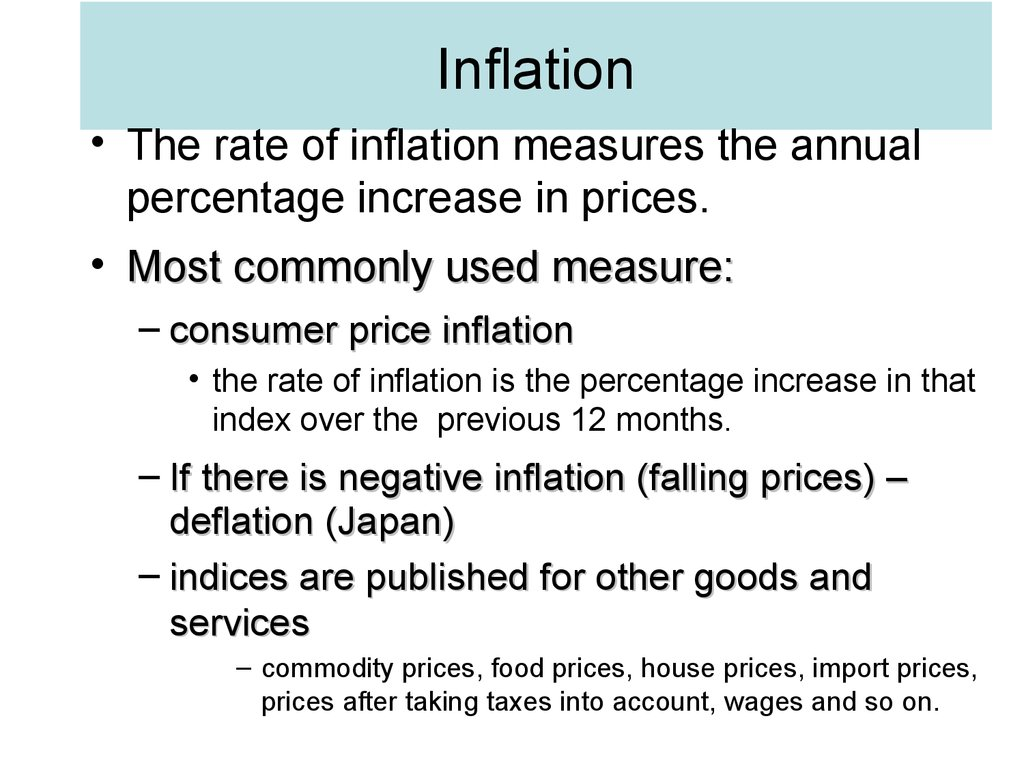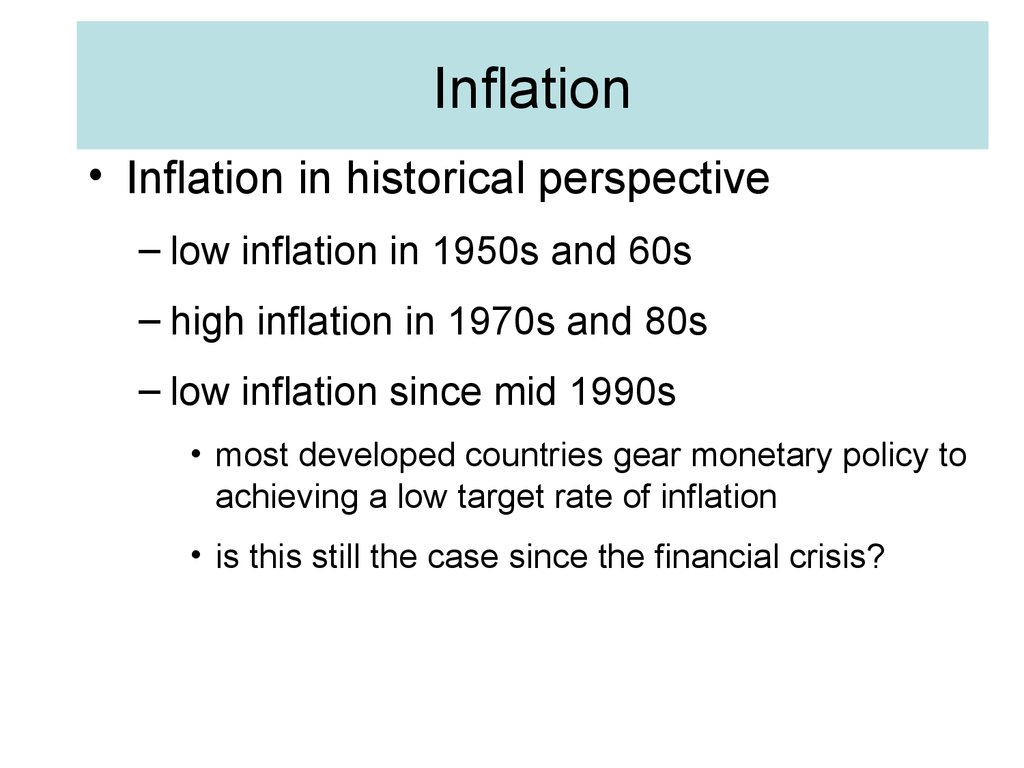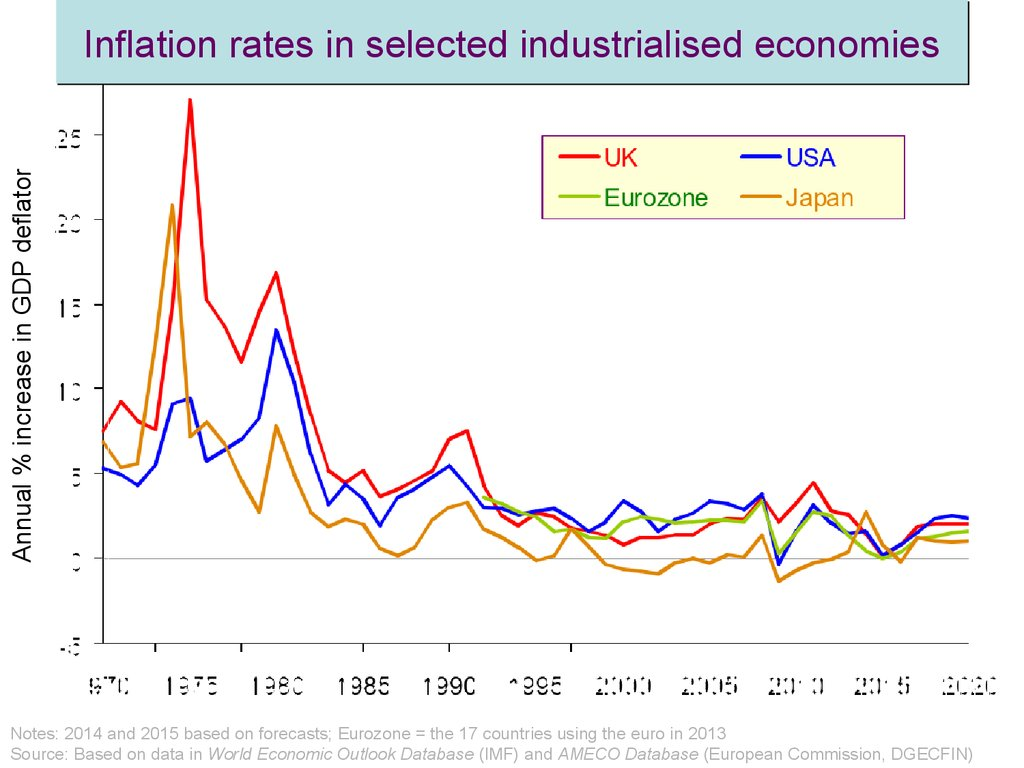# Inflation. The rate of inflation measures the annual percentage increase in prices

## 1. Inflation

• The rate of inflation measures the annual
percentage increase in prices.
• Most commonly used measure:
– consumer price inflation
• the rate of inflation is the percentage increase in that
index over the previous 12 months.
– If there is negative inflation (falling prices) –
deflation (Japan)
– indices are published for other goods and
services
– commodity prices, food prices, house prices, import prices,
prices after taking taxes into account, wages and so on.

## 2. Inflation

• Inflation in historical perspective
– low inflation in 1950s and 60s
– high inflation in 1970s and 80s
– low inflation since mid 1990s
• most developed countries gear monetary policy to
achieving a low target rate of inflation
• is this still the case since the financial crisis?

## 3. Inflation rates in selected industrialised economies

Annual % increase in GDP deflator
Inflation rates in selected industrialised economies
Notes: 2014 and 2015 based on forecasts; Eurozone = the 17 countries using the euro in 2013
Source: Based on data in World Economic Outlook Database (IMF) and AMECO Database (European Commission, DGECFIN)

## 4. The distinction between real and nominal values.

• Nominal figures are those using current
prices, interest rates, etc. Real figures are
figures corrected for inflation.
– When there is inflation, we have to be careful in
assessing how much national output,
consumption, wages, etc. are increasing.
• GDP in money terms may have risen by 5 per cent, but
if inflation is 3 per cent, real growth in GDP will be
only 2 per cent.
• A rise or fall in inflation is different from a rise or fall in
prices.
4

## 5. Q In a period of rapid inflation which of the following would be the least desirable store of wealth?

A. Vintage wine.
B. Property
C. Money
D. Land
E. Stocks and shares]

## 6. Inflation

• Aggregate demand & supply and prices
– The level of prices in the economy is
determined by the interaction of aggregate
demand and aggregate supply.
– Aggregate demand curve shows how much
national output (real GDP) will be demanded at
each level of prices.

## 7. Aggregate demand

Why the AD curve slopes downwards
• lower price level leads to higher domestic goods
consumption and fewer imports
Price level
• higher price level tends to cause interest rates to
rise: at a given level of Ms, increase in Md leads to
higher interest rates, which discourage investment
and encourage saving.
• price rise erodes value of people’s savings – they
save more to compensate.
O
National output

## 8. Inflation

• Aggregate demand & supply and prices
– aggregate demand curve
– aggregate supply curve
• The aggregate supply curve slopes upwards – at
least in the short run. In other words, the higher the
level of prices, the more will be produced.

## 9. Aggregate demand and aggregate supply

Price level
AS
If aggregate demand exceeds
aggregate supply (e.g. by a – b
at a price level of P2), price will
rise to the equilibrium level, Pe
Pe
P2
b
a
O
National output

## 10. Inflation

• Aggregate demand & supply and prices
– aggregate demand curve
– aggregate supply curve
• why AS curves generally slope upwards
– equilibrium
– shifts in AD and AS curves
• AD: if there is a change in any of its components
• AS: if there is a rise in labour productivity or capacity
of the economy

## 11. Q As the price level in the economy rises, which of the following occurs? (i) The quantity of ‘real money’ decreases; (ii) Real

aggregate demand decreases;
(iii)Total spending in money terms decreases.
A. (i) only
B. (ii) only
C. (i) and (ii)
D. (i) and (iii)
E. (i), (ii) and (iii)

## 12. Inflation

• Causes of inflation
– demand pull
• When the AD curve shifts to the right, output will rise
and unemployment may fall as a result.
• However, at the same time, prices will rise.
• Firms will respond to the rise in AD partly by raising
prices (caused by costs rise as a result of increasing
output), and partly by increasing output (there is a move
upwards along the AS curve).
– Demand pull inflation is caused by continuing
rises in aggregate demand.

Price level
AS
P1
O
Q1
National output

Price level
AS
P1
O
Q1
National output

## 15. Demand-pull inflation

Price level
AS
A rise in aggregate demand
causes a rise in the price level
(and also a rise in real GDP)
P2
P1
O
Q1
Q2
National output

## 16. Inflation

• Causes of inflation
– cost push inflation
• is associated with continuing rises in costs and
hence continuing leftward (upward) shifts in the AS
curve.
• Such shifts occur when costs of production rise
independently of aggregate demand.
• If firms face a rise in costs, they will respond partly
by raising prices and passing the costs on to the
consumer, and partly by cutting back on production.

## 17. Inflation

• Rise in costs may come from:
– wage push
• increase in wages due to trade unions activity
independently of demand for labour
– profit push
• firms use their monopoly power to make bigger profits by
pushing up prices independently of consumer demand
– import-price push
• prices rising independently of the level of AD (e.g. OPEC
putting up oil prices)
17

## 18. Inflation

• In all these cases, inflation occurs
because one or more groups are
exercising economic power.
– The problem is likely to get worse, therefore,
if there is an increasing concentration of
economic power over time (e.g. if firms or
unions get bigger and bigger, and more
monopolistic) or if groups become more
militant.
18

## 19. Q Which one of the following would be the cause of cost-push inflation?

A. A cut in the rate of income tax.
B. A cut in the rate of VAT
C. A cut in interest rates
D. A rise in the exchange rate
E. A rise in the price of oil

Price level
AS1
P1
O
Q1
National output

AS1
Price level
AS2
P1
O
Q1
National output

## 22. Cost-push inflation

Price level
AS2
AS1
A rise in costs causes a rise
in the price level
(but also a fall in real GDP)
P2
P1
O
Q2
Q1
National output

## 23. Inflation

• With the growth in demand for raw materials
and food (China, India, Brazil) rising costs
became more of a problem
• Thus, what starts with a rise in aggregate
demand in these countries (demand-pull
inflation), becomes cost-push inflation for
other countries due to globalisation,
having to pay higher prices for the
commodities they import.
23

## 24. Inflation

• Demand-pull and cost-push inflation can
occur together
– since wage and price rises can be caused
both by increases in aggregate demand
• as well as by independent causes pushing up
costs.
– Even when an inflationary process starts as
either demand-pull or cost-push, it is often
difficult to separate the two.
24

Price level
AS1
P1
O
National output

Price level
AS2
AS1
P2
P1
O
National output

AS3 AS
Price level
2
AS1
P3
P2
P1
O
National output

## 28. Inflation

• Expectations and inflation
– Workers and firms take account of the
expected rate of inflation when making
decisions.
• The employers will be happy to pay a wage rise
somewhat below 5 per cent.
• After all, they can put their price up by 5 per
cent
– Realisation of expectations

29

## 30. Aims of this session:

• Add Government spending and foreign
aggregate demand
• Explain what we mean by fiscal and
monetary policy
• Show how fiscal and monetary policy
may affect aggregate demand
30

## 31. Fiscal policy

• Fiscal policy is the government’s
• Automatic stabilisers reduce
fluctuations in aggregate demand
31

32

## 33. Government and aggregate demand 1

• Government purchases (G) of final output add
directly to aggregate demand:
AD= C + I + G
• The level of government demand reflects how
many hospitals the government wants to build,
how large it wants defence spending to be, and
so on.
33

## 34. Government and aggregate demand 2

Government levies taxes and pays out transfer
benefits
– Tax revenue and benefit spending both vary with output.
How do taxes affect disposable income?
– Assume net taxes NT = tY
• where t is the net tax rate
Households’ disposable income YD is now:
YD = Y(1-t)
34

## 35. The open economy: foreign trade and output determination

Introducing exports (X) & imports (Z)
– the value of net exports (X - Z)
– when imports exceed exports
– when exports exceed imports
• Aggregate demand
AD = C + I + G + X – Z
In equilibrium AD is equal to output and income
35

36

## 37. Monetary Policy I

• Interest rates are the instrument of monetary
policy
• The monetary instrument is the variable over
which a central bank exercises day-to-day
control
37

## 38. Monetary Policy II

Monetary policy is the decision by the Central
Bank about what the interest rate to set.
– In the UK, the central bank is the Bank of England,
which acts on behalf of the government. It has
operational independence from the government to
set interest rates.
– But the Chancellor has decided the Bank’s ultimate
objective is to set interest rates to try to keep
inflation close to 2 per cent a year
38

## 39. Nominal vs Real Interest Rates

• The real interest rate is the difference
between the nominal interest rate and
inflation
39

40

## 41. How interest rates affect the economy

Interest rates influence:
• personal consumption by changing the
cost of borrowing for consumption
• investment demand by raising the
opportunity cost of capital
41

## 42. Interest rates and investment demand

• For a given rate of
output growth the
investment demand
schedule II shows
how a lower interest
rate raises
investment demand
– If the interest rate
rises from r0 to r1
desired investment
falls from I0 to I1
42

## 43. Lower interest rates increase aggregate demand 1

• Lower interest rates induce an increase in personal
consumption and investment demand increasing AD
to AD1 and output to Y1
• Equivalently, lower interest rates increase desired
investment at any output
• but also, by reducing desired consumption, they
raise the desire to save at any output
43

## 44. Demand management and the policy mix

• Demand management is the use of monetary and fiscal
policy to stabilize output near the level of potential
output.
• The government can use fiscal and monetary policy to
control demand
• loose fiscal policy can be used with tight monetary
policy or vice versa
• the former suggests a large public sector; the latter a
smaller public sector
• the mix of policies affects the composition of output
44